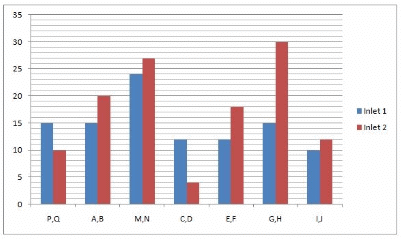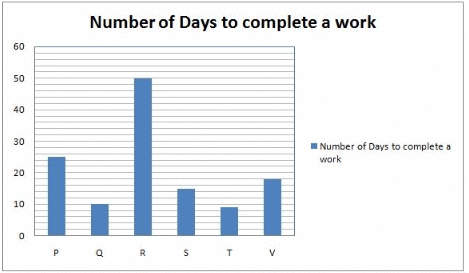# Data Interpretation MCQ -2

## 20 Questions MCQ Test IBPS PO Prelims- Study Material, Online Tests, Previous Year | Data Interpretation MCQ -2

Description
Attempt Data Interpretation MCQ -2 | 20 questions in 40 minutes | Mock test for Quant preparation | Free important questions MCQ to study IBPS PO Prelims- Study Material, Online Tests, Previous Year for Quant Exam | Download free PDF with solutions
QUESTION: 1

### Study the following graph carefully to answer the given questions Time taken by the pipes to fill a tank/cistern (hours/minutes)A large cistern can be filled by two pipes P and Q. How many minutes will it take to fill the Cistern from an empty state if Q is used for half the time and P and Q fill it together for the other half?

Solution:

Part filled by P and Q = 1/15 + 1/10 = 1/6
Part filled by Q = 1/10
x/2(1/6 + 1/10) = 2/15 = 15/2 = 7.5 minutes

QUESTION: 2

### Study the following graph carefully to answer the given questions Time taken by the pipes to fill a tank/cistern (hours/minutes)Two pipes M and N can fill a tank. If both the pipes are opened simultaneously, after how much time should N be closed so that the tank is full in 8 minutes?

Solution:

Required time = y(1-(t/x)) = 27(1-(8/24))= 18 minutes

QUESTION: 3

### Study the following graph carefully to answer the given questions Time taken by the pipes to fill a tank/cistern (hours/minutes)Three pipe E, F, and R can fill a tank. If Pipe R alone can fill a tank in 24 minutes then the pipe R is closed 12 minutes before the tank is filled. In what time the tank is full?

Solution:

Let T is the time taken by the pipes to fill the tank
(1/12 + 1/18 + 1/24)*(T – 12) + (1/12 + 1/18)*12 = 1
We will get T = 108/13 = 8.(4/13)

QUESTION: 4

Study the following graph carefully to answer the given questions

Time taken by the pipes to fill a tank/cistern (hours/minutes)Two pipes C and D can fill a cistern. If they are opened on alternate minutes and if pipe C is opened first, in how many minutes will the tank be full?

Solution:

Pipe P can fill = 1/12
Pipe Q can fill = 1/4
For every two minutes, 1/12 + 1/4 = 1/3 Part filled
Total = 6 minutes

QUESTION: 5

Study the following graph carefully to answer the given questions

Time taken by the pipes to fill a tank/cistern (hours/minutes)Two pipes, A and B are opened simultaneously and it is found that due to the leakage in the bottom, 17/7 minutes are taken extra to fill the tank. If the tank is full, in what approximate time would the leak empty it?

Solution:

Total time taken by both pipes before the leak was developed = 60/7 minutes
now, leaks is developed which will take T time to empty the tank so, (1/15 +1/20 – 1/T) = 1/11
solve for T, we will get 660/17 minutes = 39 minutes (approx.)

QUESTION: 6

Study the following graph carefully to answer the given questions

Time taken by the pipes to fill a tank/cistern (hours/minutes)A waste pipe, W can carry off 12 litre of water per minute. If all the pipes I, J and W are opened when the tank is full and it takes one hour to empty the tank. Find the capacity of the tank.

Solution:

Let the waste pipe take ‘T’ time to empty the tank.
(1/10 + 1/12 – 1/T)*60 = -1
We will get T = 5 min
So capacity = 5*12 = 60ltr

QUESTION: 7

Study the following graph carefully to answer the given questions

Time taken by the pipes to fill a tank/cistern (hours/minutes)Two pipes G, and H are opened and when the tank is 1/3 full a leak is developed due to which 1/3 water supplied by the pipe leaks out. What is the total time to fill the tank?

Solution:

(1/15 + 1/30)*T1 = 1/3, T1 = 10/3 minutes
Now after leak is developed, [(1/15 + 1/30) – (1/3)*(1/15 + 1/30)]*T2 = 2/3
T2 = 10 minutes. So total time = 10 + 10/3 = 40/3 minutes

QUESTION: 8

Study the following graph carefully to answer the given questions

Time taken by the pipes to fill a tank/cistern (hours/minutes)Two pipes E and F are opened simultaneously and it is found that due to leakage in the bottom of the tank it took 48 minutes excess time to fill the cistern. When is the cistern full, in what time will the leak empty it?

Solution:

Work done by the two pipes in 1 hour = (1/12)+(1/18) = (15/108).
Time taken by these pipes to fill the tank = (108/15)hrs = 7 hours 12 min.
Due to leakage, time taken = 7 hours 12 min + 48 min = 8 hours
Work done by two pipes and leak in 1 hour = 1/8.
Work done by the leak in 1 hour =(15/108)-(1/8)=(1/72).
Leak will empty the full cistern in 72 hours.

QUESTION: 9

Study the following graph carefully to answer the given questions

Time taken by the pipes to fill a tank/cistern (hours/minutes)Three pipes C, A and B can fill a tank. If pipe C is opened all the time and pipe A and B are opened for one hour alternatively. The tank will be full in

Solution:

(1/12 + 1/15) + (1/12 + 1/20) = 17/60 (in 2 hrs this much tank is filled)
so in 6 hrs 51/60 is filled. Remaining, 9/60 = (1/12 + 1/15)*t, so T = 1hr
so total = 6 + 1 = 7 hr

QUESTION: 10

Study the following graph carefully to answer the given questions

Time taken by the pipes to fill a tank/cistern (hours/minutes)Three pipes R, P and Q can fill the tank. R can fill the tank 5 minutes less than that of Q. If all the pipes are opened together and pipe P is turned off 5 minutes before the tank is filled. Then find the time in which the tank will full.

Solution:

Let total time taken by the pipes is T hrs, then
(1/5 + 1/10 + 1/15)*(T – 5) + (1/5 + 1/15)*5 = 1

QUESTION: 11

Study the following graph carefully to answer the given questionsP and R started the work jointly. A few days later U also joined them and thus all of them completed the whole work in 10 days. All of them were paid total Rs.600. What is the Share of U?

Solution:

Efficiency of P = 4%
Efficiency of R = 2%
[(4+2)*10] = 60%
The remaining work was done by U = 40%.
40% of 600 = 240

QUESTION: 12

Study the following graph carefully to answer the given questionsQ and S work together for 5 days, the rest of the work is finished by M in two more days. If they get Rs. 6000 as wages for the whole work, what are the daily wages of Q, S and M respectively?

Solution:

Explanation:
Q’s 5 days work = 50%
S’s 5 days work = 33.33%
M’s 2 days work = 16.66% [100- (50+33.33)] Ratio of work of Q, S and M = 3: 2: 1
Q’s total share = Rs. 3000
S’s total share = Rs. 2000
M’s total share = Rs. 1000
Q’s one day’s wage = Rs.600
S’s one day’s wage = Rs.400
M’s one day’s wage = Rs.500

QUESTION: 13

Study the following graph carefully to answer the given questionsThe efficiency of L is 25% more than P. L started a work alone and then P joined her 5 days before actual completion of the work. For how many days L worked alone?

Solution:

Efficiency (L : P) = 5 : 4
Number of days(L : P) = 4x : 5x = 4x : 25
∴ Number of days required by L to finish the work alone = 4x
= 4 x 5 = 20.
L and P work together for last 5 days = 5 x 9 = 45%
Efficiency of L = 5% and P’s efficiency = 4%
∴ No. of days taken by L to complete 55% work = 55/5 = 11days

QUESTION: 14

Study the following graph carefully to answer the given questionsR started the work and left after some days, when 25% work was done. After it Z joined and completed it working for 25 days. In how many days R and Z can do the complete work, working together?

Solution:

Efficiency of R = (100/50) = 2%
Rest work = 75%
∴ Efficiency of Z = 75/25 = 3%
∴ Combined efficiency of R and Z = 5%
∴ Number of days required by R and Z to work together = 100/5 = 20 days.

QUESTION: 15

Study the following graph carefully to answer the given questionsT and V started the work. After 3 days Z joined them, who can complete alone the same whole work in 3 days. What is the total number of days in which they had completed the work?

Solution:

Efficiency of T and V = 11.11 + 5.55 = 16.66%
Work done in 3 days = 3 x 16.66 = 50%
Rest work done by T, V and Z = 50/50 = 1 day
Work can be completed in 4 days.

QUESTION: 16

Study the following graph carefully to answer the given questionsA is twice efficient as B and together they do the same work in as much time as T and V together. In how many days A can complete the work individually?

Solution:

1/x + 1/2x = 1/9 + 1/18
3/2x = 3/18
Number of days taken by A = 9 days

QUESTION: 17

Study the following graph carefully to answer the given questionsAfter working for 3 days S is joined by O. If they complete the remaining work in 3 more days, in how many days can O alone complete the work?

Solution:

Total days S worked = 3+3 = 6
6/15 = 2/5
So 3/5 = 3/x
x = 5

QUESTION: 18

Study the following graph carefully to answer the given questionsM can do a piece of work 5 days less than that of P. M and P began to work together but M leaves after some days and P completed the remaining work in 12 days. Number of days after which M left the job-

Solution:

(1/20 + 1/25)*T + 12/25 = 1
We will get T = 52/9 i.e. 5.7/9 days

QUESTION: 19

Study the following graph carefully to answer the given questionsX can do a certain work in the same time in which Y and R together can do it. If X and Y together could do it in the same time as that of Q then Y alone could do it in:

Solution:

X, Y and R’s 1 day work = 1/10 + 1/50 = 6/50 = 3/25
X’s 1 day work = Y + R‘s 1 day work
2*( x’s 1 day work) = 3/25
x’s 1 day work = 3/50
Y’s 1 day work = 1/10 – 3/50 = 2/50 = 1/25

QUESTION: 20

Study the following graph carefully to answer the given questionsA can do a piece of work 10 days more than that of Q. Both of them start the work but A leaves the work 5 days before its completion. Find the time in which work is completed

Solution:

(1/10 + 1/20)*(T-5) + 5/20 = 1 (T is the number of days in which the work is completed)Use Code STAYHOME200 and get INR 200 additional OFF Use Coupon Code